# Twisted BeamThis test lets you check analysis results for a twisted beam, in the context of a static case.

You can use either the 2D or 3D model.Reference:

R. MAC NEAL, R.L. HARDER., A Proposed Standard Set of Problems to Test Finite Element Accuracy, Finite Element Analysis Design, Vol. 1, P.3-20, 1985.

##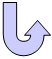Specifications

### Geometry Specifications

 Length: L = 12 m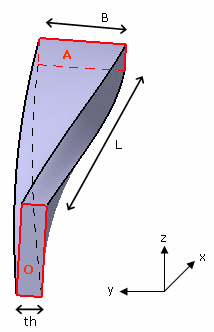Width: B = 1.1 m Thickness: th =  320 mm Rotation: 90 degrees between O and A

### Analysis Specifications

 Young Modulus (material): E = 29 MPa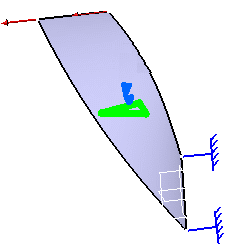Poisson's ratio (material): ν = 0.22 Mesh Specifications: Size = 275 mm Restraints: Clamp on section O Loads (surface force density): Two loads are applied on the beam section at A.  Pz = 1 N or Py = 1 N

##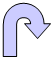Results

• Analytical Results:
• For Pz = 1, Tz = 1.75 mm
• For Py = 1, Ty = 5.42 mm
• The results correspond to the displacement T in the direction of the load at section A.
Normalized results (computed results divided by analytical solution) are listed.

 Load Quantity Linear triangle (TR3) Parabolic triangle (TR6) Linear quadrangle (QD4) Parabolic quadrangle (QD8) Linear tetrahedron (TE4) Parabolic tetrahedron (TE10) Linear hexahedron (HE8) Parabolic hexahedron (HE20) Linear pentahedron (WE6) Parabolic pentahedron (WE15) Pz = 1 Tz [mm] 2.842 1.751 1.752 1.75 0.83 1.749 1.75 1.77 1.72 1.75 Normalized Results 1.62 1.00 1.00 1.00 0.47 1.00 1.00 1.011 0.929 1.00 Py = 1 Ty [mm] 9.806 5.416 5.416 5.418 2.352 5.422 5.43 5.47 5.34 5.41 Normalized Results 1.81 1.00 1.00 1.00 0.43 1.00 1.002 1.009 0.985 0.998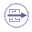To Perform the Test:

The Twisted_beam_TE10.CATAnalysis document presents a complete analysis, computed with a mesh formed of parabolic tetrahedron elements (TE10).

The Twisted_beam_TR6.CATAnalysis document presents a complete analysis, computed with a mesh formed of parabolic triangle elements (TR6).

The twistedbeamWE15.CATAnalysis document presents a complete analysis, computed with a mesh formed of parabolic pentahedron elements (WE15).
The mesh of 3D elements is obtained using the Sweep 3D mesher: the guides are given by the beam length.

To compute the case with linear elements, proceed as follow:

1. Open one of the CATAnalysis documents.

2. In the Advanced Meshing Tools workbench, replace the mesh specifications as indicated above.

3. In the Generative Structural Analysis workbench, compute the case.